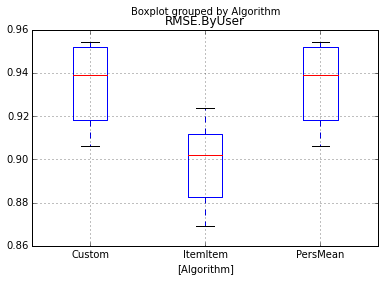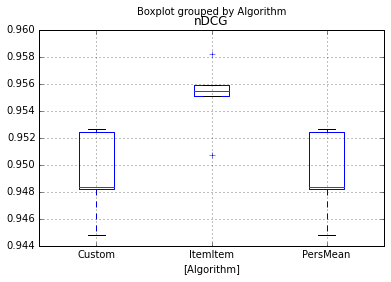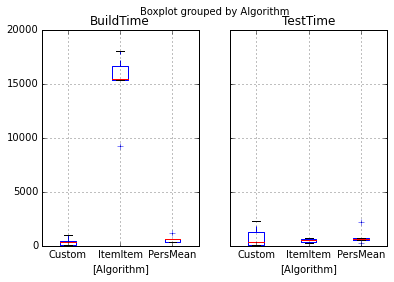# Analyze Results from Evaluation Quickstart¶

This notebook analyzes the results of the evaluation quickstart.

## Setup¶

First, we need to import our libraries:

In :
import pandas as pd
import matplotlib

In :
%matplotlib inline


## Data Import and Preparation¶

LensKit puts its output in a csv file:

In :
results = pd.read_csv('build/eval-results.csv')

Out:
Algorithm DataSet Partition BuildTime TestTime RMSE.ByUser RMSE.ByRating nDCG
0 PersMean ML100K 2 670 250 0.918045 0.960007 0.952628
1 PersMean ML100K 0 361 586 0.951878 0.947020 0.948389
2 PersMean ML100K 3 360 721 0.954364 0.922529 0.944793
3 PersMean ML100K 1 648 574 0.938969 0.981708 0.948190
4 Custom ML100K 3 340 375 0.954364 0.922529 0.944793

We ran each algorithm 5 times since we used 5-fold cross-validation. What we want to do next is compute the average value of each metric for each data set.

In :
agg_results = results.drop(['Partition'], axis=1).groupby('Algorithm').mean()
agg_results

Out:
BuildTime TestTime RMSE.ByUser RMSE.ByRating nDCG
Algorithm
Custom 404.2 815.0 0.933897 0.948108 0.949287
ItemItem 14934.4 488.8 0.897912 0.904838 0.955086
PersMean 646.0 875.6 0.933897 0.948108 0.949288

## Plotting Results¶

Let's start plotting things. What's the RMSE achieved by each algorithm?

In :
results.loc[:,['Algorithm', 'RMSE.ByUser']].boxplot(by='Algorithm')

Out:
<matplotlib.axes._subplots.AxesSubplot at 0xb062b70>Next up: nDCG

In :
results.loc[:,['Algorithm', 'nDCG']].boxplot(by='Algorithm')

Out:
<matplotlib.axes._subplots.AxesSubplot at 0xb06ed30>Finally, the build and test times.

In :
results.loc[:,['Algorithm', 'BuildTime', 'TestTime']].boxplot(by='Algorithm')

Out:
array([<matplotlib.axes._subplots.AxesSubplot object at 0x000000000B369198>,
<matplotlib.axes._subplots.AxesSubplot object at 0x000000000B16A8D0>], dtype=object)In :